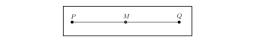# The midpoint 2

Find the value of x if M is the midpoint of PQ, PQ=10x−7, and PM=14.

x =  3.5

### Step-by-step explanation:Did you find an error or inaccuracy? Feel free to write us. Thank you!

Tips for related online calculators
Looking for help with calculating arithmetic mean?
Looking for a statistical calculator?
Do you have a linear equation or system of equations and looking for its solution? Or do you have a quadratic equation?
Do you want to convert length units?

#### Grade of the word problem:

We encourage you to watch this tutorial video on this math problem: